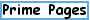Reference Database (references for the Prime Pages)Home
Search Site

Largest
Finding
How Many?
MersenneGlossary

Prime Curios!
e-mail list

FAQ
Prime Lists
Titans

Submit primes
This is the Prime Pages' interface to our BibTeX database.  Rather than being an exhaustive database, it just lists the references we cite on these pages.  Please let me know of any errors you notice.
References: [ Home | Author index | Key index | Search ]

#### Item(s) in original BibTeX format

@article{BR98,
author={A. Bj{\"o}rn and H. Riesel},
title={Factors of Generalized {Fermat} Numbers},
abstract={A search for prime factors of the generalized Fermat numbers $F_n(a,b)=a^{2^n}+b^{2^n}$
has been carried out for all pairs $(a,b)$ with $a,b\leq 12$ and $\gcd(a,b)=1$.
The search $k$ limit on the factors, which all have the form $p=k \cdot 2^m+1$, was $k=10^9$ for $m\leq 100$ and $k=3 \cdot 10^6$ for $101\leq m \leq 1000$. Many larger primes of this form have also been tried as factors
of $F_n(a,b)$. Several thousand new factors were found, which are given
in our tables. For the smaller of the numbers, i.e. for $n\leq 15$, or,
if $a,b\leq 8$, for $n\leq 16$, the cofactors, after removal of the factors
found, were subjected to primality tests, and if composite with , searched
for larger factors by using the ECM, and in some cases the MPQS, PPMPQS,
or SNFS. As a result all numbers with $n \leq 7$ are now completely factored.},
journal= mc,
volume= 67,
year= 1998,
pages={441--446},
mrnumber={98e:11008}
}Another prime page by Reginald McLean